## Optimal Unit Commitment (UC):

As is evident, it is not economical to run all the units available all the time. To determine the units of a plant that should operate for a particular load is the problem of unit commitment (UC). This problem is of importance for thermal plants as for other types of generation such as hydro; their operating cost and start-up times are negligible so that their on-off status is not important.

A simple but sub-optimal approach to the problem is to impose priority ordering, wherein the most efficient unit is loaded first to be followed by the less efficient units in order as the load increases.

A straightforward but highly time-consuming way of finding the most economical combination of units to meet a particular load demand, is to try all possible combinations of units that can supply this load; to divide the load optimally among the units of each combination by use of the coordination equations, so as to find the most economical operating cost of the combination; then, to determine the combination which has the least operating cost among all these. Considerable computational saving can be achieved by using branch and bound or a dynamic programming method for comparing the economics of combinations as certain combinations Meet not be tried at all.

### Dynamic Programming Method:

In a practical problem, the UC table is to be arrived at for the complete load cycle. If the load is assumed to increase in small but finite size steps, dynamic programming (DP) can be used to advantage for computing the UC table, wherein it is not necessary to solve the coordination equations; while at the same time the unit combinations to be tried are much reduced in number. For these reasons, only the DP approach will be advanced here.

The total number of units available, their individual cost characteristics and the load cycle on the station are assumed to be known a priori. Further, it shall be assumed that the load on each unit of combination of units changes in suitably small but uniform steps of size ΔMW (e.g. 1 MW).

Starting arbitrarily with any two units, the most economical combination is determined for all the discrete load levels of the combined output of the two units. At each load level the most economic answer may be to run either unit or both units with a certain load sharing between the two. The most economical cost curve in discrete form for the two units thus obtained, can be viewed as the cost curve of a single equivalent unit. The third unit is now added and the procedure repeated to find the cost curve of the three combined units. It may be noted that in this procedure the operating combinations of third and first, also third and second are not required to be worked out resulting in considerable saving in computational effort. The process is repeated, till all available units are exhausted. The advantage of this approach is that having obtained the optimal way of loading k units, it is quite easy to determine the optimal manner of loading (k + 1) units.

Let a cost function FN (x) be defined as follows:

• FN (x) = the minimum cost in Rs/hr of generating x MW by N units,
• fN (y) = cost of generating y MW by the Nth unit
• FN-1 (x – y) = the minimum cost of generating (x – y) MW by the remaining (N – 1) units

Now the application of DP results in the following recursive relation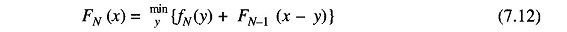Using the above recursive relation, we can easily determine the combination of units, yielding minimum operating costs for loads ranging in convenient steps from the minimum permissible load of the smallest unit to the sum of the capacities of all available units. In this process the total minimum operating cost and the load shared by each unit of the optimal combination are automatically determined for each load level.

The use of DP for solving the UC problem is best illustrated by means of an example. Consider a sample system having four thermal generating units with parameters listed in Table 7.2. It is required to determine the most economical units to be committed for a load of 9 MW. Let the load changes be in steps of 1 MW.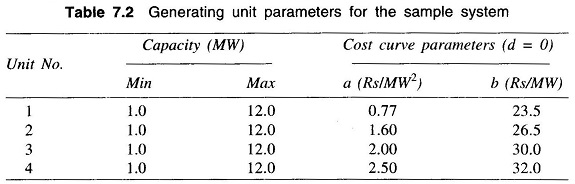Now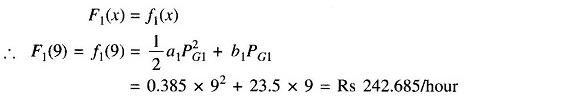From the recursive relation (7.12), computation is made for F2(0), F2(1), F2(2), …, F2(9). Of these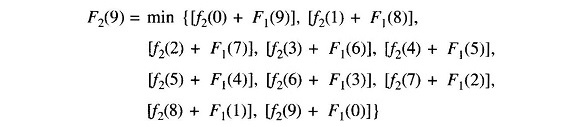On computing term-by-term and comparing, we get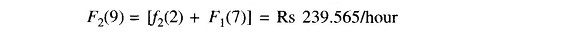Similarly, we can calculate F2(8), F2(7), …, F2(1), F2(0).

Using the recursive relation (7.12), we now compute F3(0), F3(1), …, F3(9).

Of these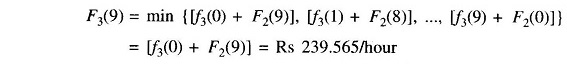Proceeding similarly, we get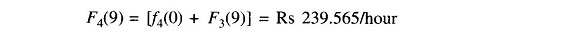Examination of F1(9), F2(9), F3(9) and F4(9) leads to the conclusion that optimum units to be committed for a 9 MW load are 1 and 2 sharing the load as 7 MW and 2 MW, respectively with a minimum operating cost of Rs 239.565/hour.

It must be pointed out here, that the optimal UC table is independent of the numbering of units, which could be completely arbitrary. To verify, the reader may solve the above problem once again by choosing a different unit numbering scheme.

If a higher accuracy is desired, the step size could be reduced (e.g.1/2 MW),with a considerable increase in computation time and required storage capacity.

The effect of step size could be altogether eliminated, if the branch and bound technique is employed. The answer to the above problem using branch and bound is the same in terms of Unit Commitment, i.e. units 1 and 2, but with a load sharing of 7.34 MW and 1.66 MW, respectively and a total operating cost of Rs 239.2175/hour.

In fact the best scheme is to restrict the use of the DP method to obtain the UC table for various discrete load levels; while the load sharing among Unit Commitment is then decided by use of the coordination Eq. (7.10).

For the example under consideration, the UC table is prepared in steps of 1 MW. By combining the load range over which the Unit Commitment does not change, the overall result can be telescoped in the form of Table 7.3.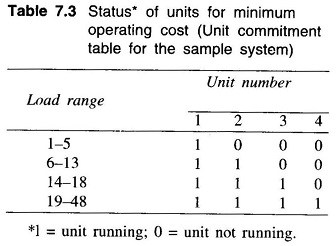The UC table is prepared once and for all for a given set of units. As the load cycle on the station changes, it would only mean changes in starting and stopping of units with the basic UC table remaining unchanged.

Using the UC table and increasing load in steps, the most economical station operating cost is calculated for the complete range of station capacity by using the coordination equations. The result is the overall station cost characteristic in the form of a set of data points. A quadratic equation (or higher order equation, if necessary) can then be fitted to this data for later use in economic load sharing among generating stations.

Scroll to Top